# Chemical Equations Writing and Balancing Equations Chemical Reactions

• Slides: 13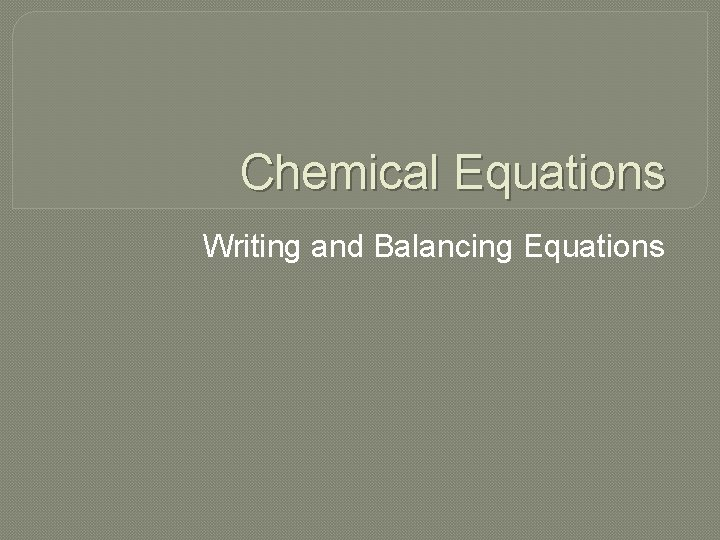Chemical Equations Writing and Balancing Equations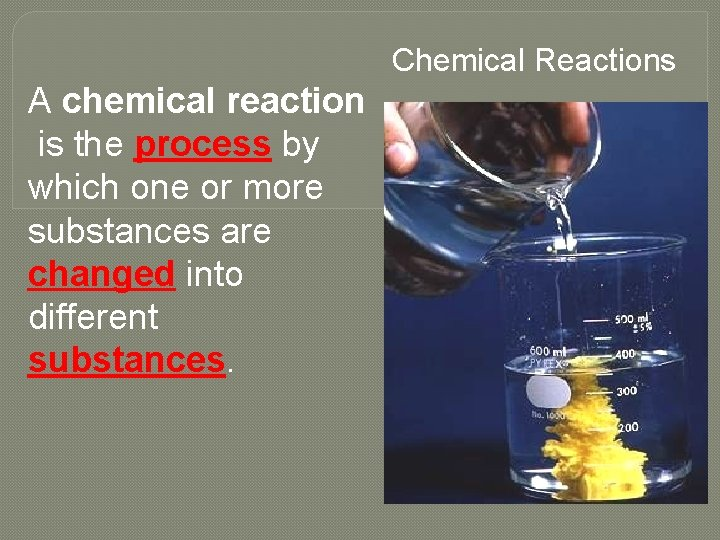Chemical Reactions A chemical reaction is the process by which one or more substances are changed into different substances.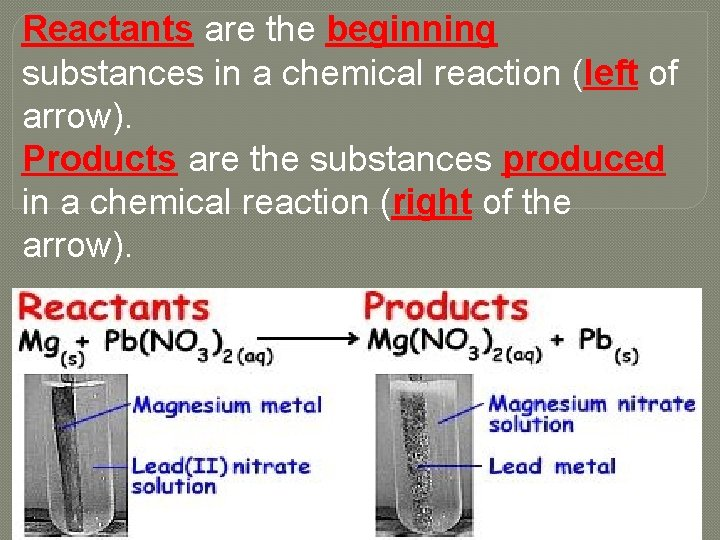Reactants are the beginning substances in a chemical reaction (left of arrow). Products are the substances produced in a chemical reaction (right of the arrow).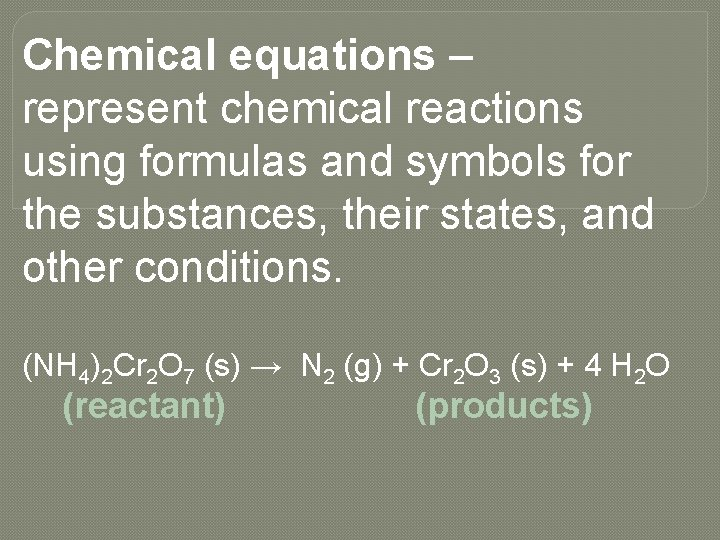Chemical equations – represent chemical reactions using formulas and symbols for the substances, their states, and other conditions. (NH 4)2 Cr 2 O 7 (s) → N 2 (g) + Cr 2 O 3 (s) + 4 H 2 O (reactant) (products)Law of Conservation of Mass – states that matter can neither be created or destroyed in a chemical reaction. It can only change forms. Antoine-Laurent de LavoisierTherefore, the same number of each kind of atom must be found on the left and on the right sides of a chemical equation. 2 H 2 + O 2 2 H 2 O This is accomplished by adding coefficients to the front of compounds and elements. Do not change subscripts.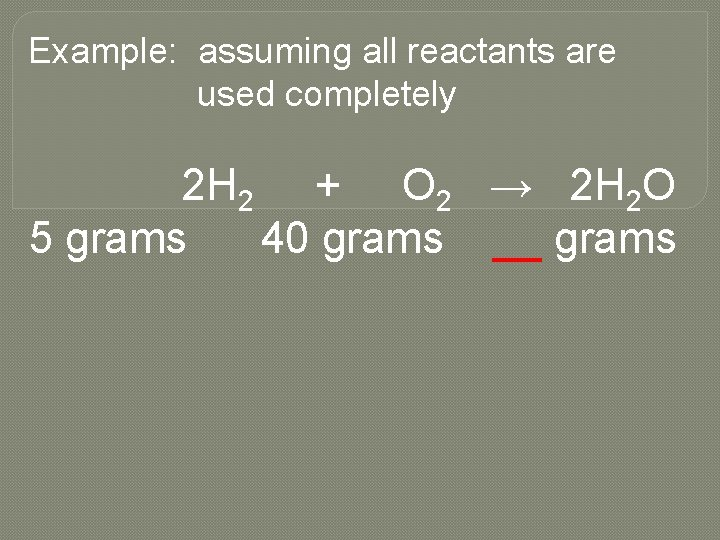Example: assuming all reactants are used completely 2 H 2 + O 2 → 2 H 2 O 5 grams 40 grams __ grams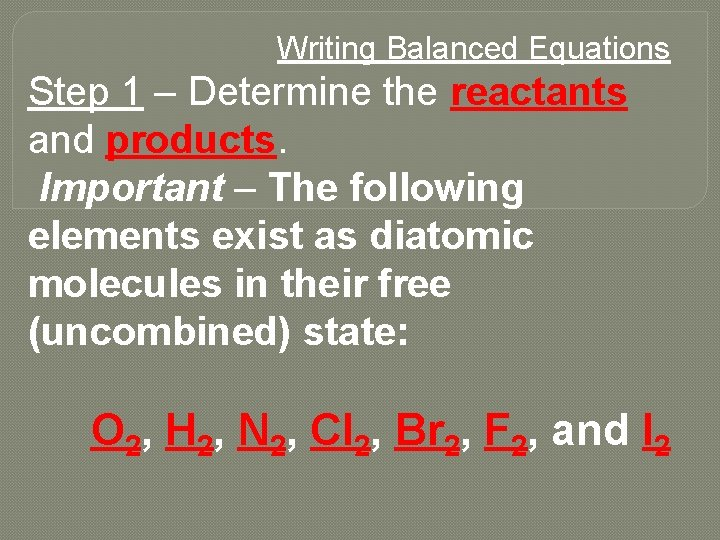Writing Balanced Equations Step 1 – Determine the reactants and products. Important – The following elements exist as diatomic molecules in their free (uncombined) state: O 2, H 2, N 2, Cl 2, Br 2, F 2, and I 2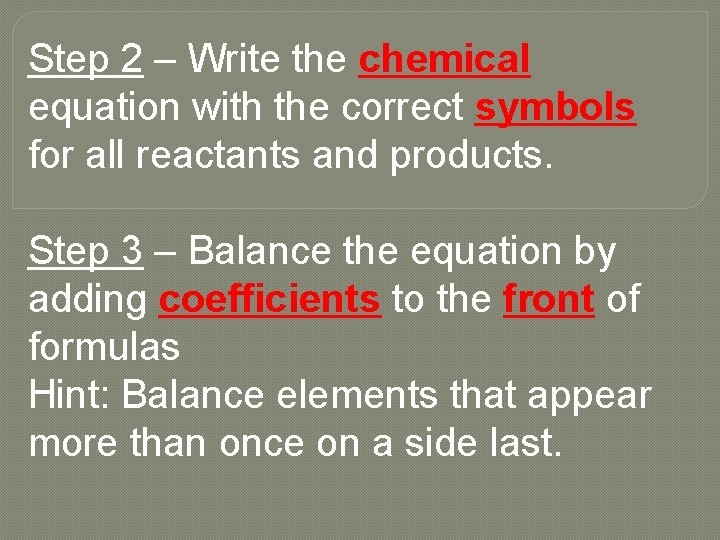Step 2 – Write the chemical equation with the correct symbols for all reactants and products. Step 3 – Balance the equation by adding coefficients to the front of formulas Hint: Balance elements that appear more than once on a side last.Step 4 – Add symbols, when appropriate. COMMON SYMBOLS àyields (separates reactants from products) heat required (this symbol is placed over the arrow) (l) liquid (aq) aqueous (dissolved in water) (g) gas ( an is sometimes used for a gas product)(s) solid (and is sometimes used for a precipitate) (cr) crystalline solid **Note: A formula over the yield arrow indicates a catalyst.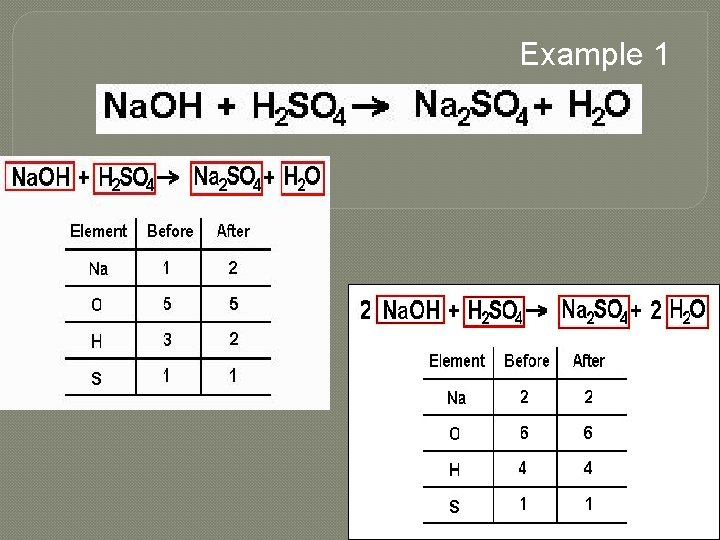Example 1Example 2 CH 3 OH (l) + O 2 (g) → CO 2 (g) + H 2 O (g) Example 3 Liquid water is formed from the combining of hydrogen and oxygen gases. (Write the Chemical Equation)